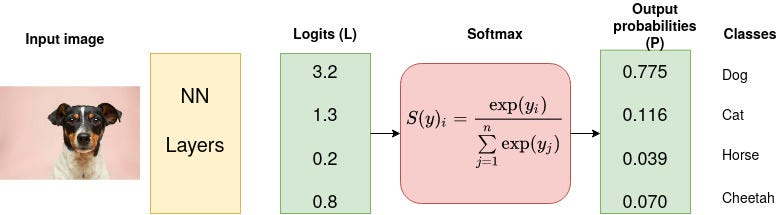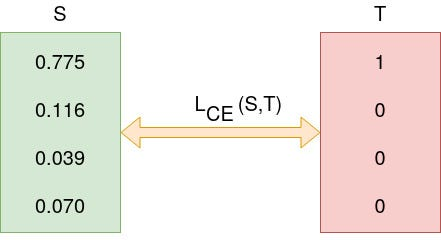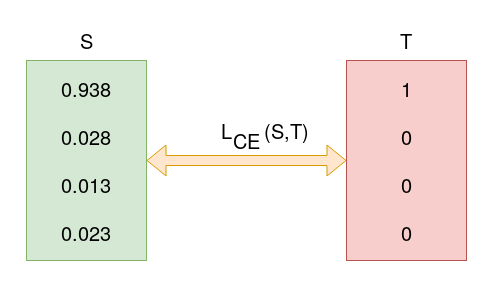# Cross Entropy

## Entropy

Entropy of a random variable X is the level of uncertainty inherent in the variables possible outcome.
$H(X) = -\sum_x p(x) \log p(x)$
Cross-Entropy loss is an important cost function. It is used to optimize classification models. The understanding of Cross-Entropy is pegged on understanding of Softmax activation function.
Consider a 4-class classification task where an image is classified as either a dog, cat, horse, or cheetah.In the above Figure, Softmax converts logits into probabilities. The purpose of the Cross-Entropy is to take the output probabilities (P) and measure the distance from the truth values (as shown in Figure below).Image for post
For the example above the desired output is [1,0,0,0] for the class dog but the model outputs [0.775, 0.116, 0.039, 0.070] .

### Cross-Entropy Loss Function

Also called logarithmic loss, log loss or logistic loss. Each predicted class probability is compared to the actual class desired output 0 or 1 and a score/loss is calculated that penalizes the probability based on how far it is form the actual expected value. The penalty is logarithmic in nature yielding a large score for large differences close to 1 and small score for small differences tending to 0. Cross-entropy is defined as,
$L_{ce} = - \sum_{i=1}^n y_i \log(p_i)$

### Categorial Cross-Entropy Loss

$L=-\sum_{i=1}^4 Y_i \log (S_i) \\ = - [1\log_2(0.775) + 0\log_2(0.116) + 0\log_2(0.039) + 0\log_2(0.070)] \\ = 0.3677$
Assume that after some iterations of model training the model outputs the following vector of logitsImage for post
$L = - 1 \log_2(0.938)+0+0+0 = 0.095$

### Binary Cross-Entropy Loss

If there are just two class labels, the probability is modeled as the Bernoulli distribution for the positive class label. This means that the probability for class 1 is predicted by the model directly, and the probability for class 0 is given as one minus the predicted probability, for example,
$L= - \sum_{i=1}^2 y_i \log(p_i) = -[y_i \log( \hat{y_i}) + (1-y_i) \log(1-\hat{y_i})]$